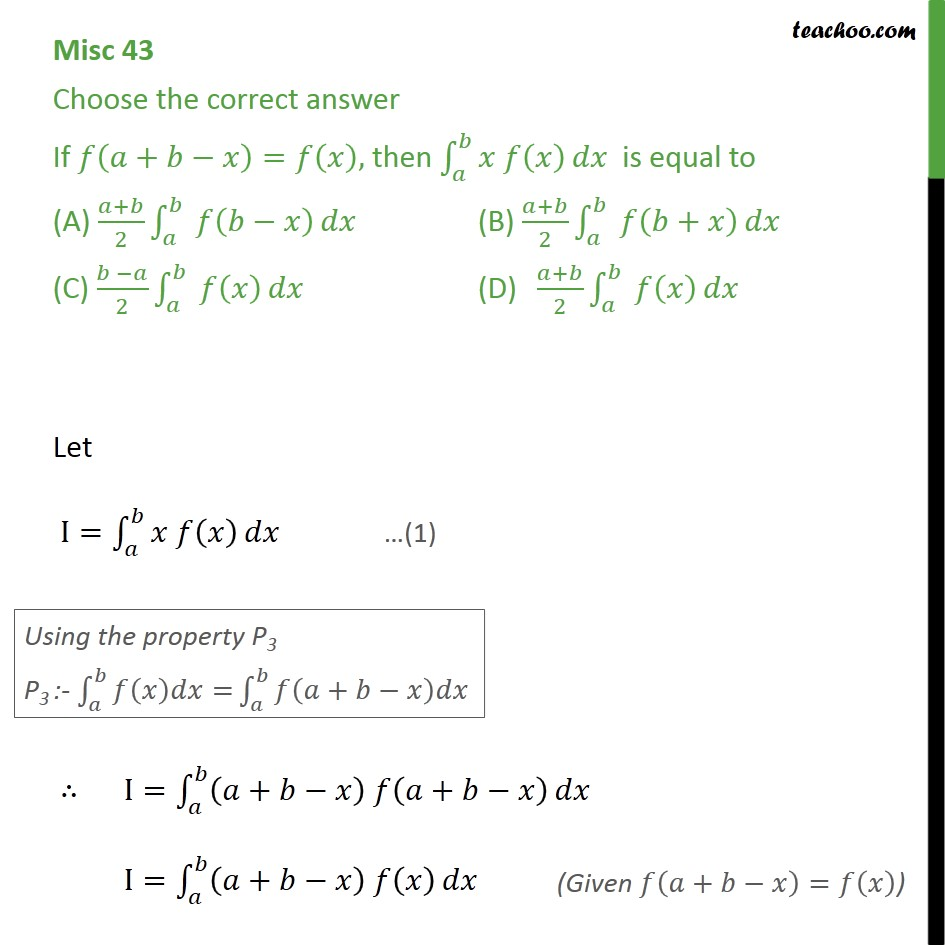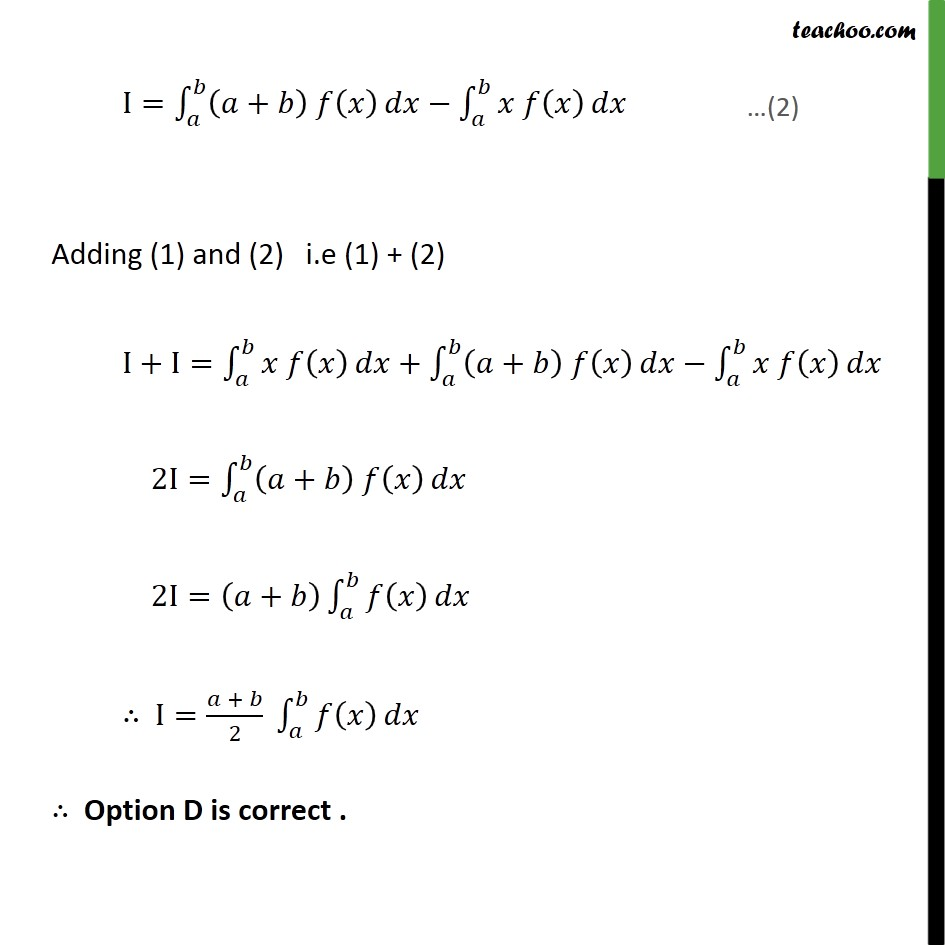Definite Integration by properties - P3

Chapter 7 Class 12 Integrals
Concept wiseSolve all your doubts with Teachoo Black (new monthly pack available now!)

### Transcript

Misc 43 Choose the correct answer If + = , then is equal to (A) + 2 (B) + 2 + (C) 2 (D) + 2 Let I= I= + + I= + I= + Adding (1) and (2) i.e (1) + (2) I+I= + + 2I= + 2I= + I= + 2 Option D is correct .